# IBPS RRB 2018 Prelims : Quants – Day 13

Dear Bankersdaily Aspirants,

IBPS RRB PO & Office Assistant 2018 Preliminary Examination starts from 11th August, 2018. The IBPS RRB Exam 2018 is one of the most expected exam of the year. The reason for the consummate expectation is different from that of the others.

The number of candidates attending the IBPS RRB PO & Office Assistant is high when compared to the other exams like SBI & Insurance Exams. The other important factor is that , no English section is there in the preliminary examination of the IBPS RRB PO & Assistant Exams. This is really a boost to the candidates as many of the aspirants couldn’t get the requisite marks in the English section and this exam is the best bet for the aspirants.

Since only few days are left for the IBPS RRB PO & Assistant Exam 2018 Preliminary Exam , we will be providing daily 20 Questions in Quantitative Aptitude & Reasoning Section to evoke the preparation mode.

Aspirants can answer the Questions by commenting the answers for the Questions in the comments section. We will be providing the answers for the Questions @ 6:00 P.M everyday.

## IBPS RRB PO & Assistant 2018 – Prelims Exam – Day 13

Topic : Quantitative Aptitude

Total Number of Questions : 20

Total Time : 15 minutes

D.21-25) Solve the equations and give the relationship between x and y.

Q.1)

I. 6x2 + 4x = 2

II.Y2 + 14y = -48

a) X > Y

b) X < Y

c) X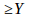d)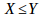e) X = Y or the relationship cannot be determined.

Q.2)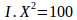II. Y =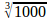a) X > Y

b) X < Y

c) Xd)e) X = Y or the relationship cannot be determined.

Q.3)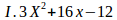= 0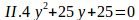a) X > Y

b) X < Y

c) Xd)e) X = Y or the relationship cannot be determined.

Q.4)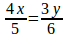a) X > Y

b) X < Y

c) Xd)e) X = Y or the relationship cannot be determined.

Q.5)

I. 17X – 32Y = -58

II. 12X + 14Y = 142.

a) X > Y

b) X < Y

c) Xd)e) X = Y or the relaationship cannot be determined.

Q.6) A alone can do a piece of work in 20 days. B alone can do the same work in 2x days. C alone can do the same work in 40 days. If all of them together can do the same work in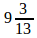days, then find the value of x.

a) 15

b) 40

c) 60

d) 26

e) 30

Q.7) A bag contains 3 white balls, 4 green balls and 5 yellow balls. If three balls are taken at random, what is the probability that three balls are green?

a)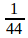b)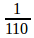c)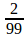d)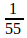e)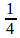Q.8) Train A travels at the speed of 40 km/hr and it takes 18 seconds to pass a platform. If the length of the train is 360 m, then find the length of the platform.

a) 240 m

b) 200 m

c) 180 m

d) 120 m

e) 160 m

Q.9) Man A is running at the speed of 30 km/hr. Man B is running at the speed of x km/hr. Man A and Man B started from same place and same time, If after 5 hours, man A covers 50 km more than man B, then find the speed of the man B.

a) 10 km/hr

b) 20 km/hr

c) 30 km/hr

d) 25 km/hr

e) 15 km//hr

Q.10) A invested Rs.30000 in bank A at the interest rate of 20% at C.I per annum. How much amount does he received after 3 years?

a) Rs.52480

b) Rs.54350

c) Rs.51840

d) Rs.50480

e) Rs.52250

Q.11) Average age of 12 boys is 30 years, if average age of the first 7 boys is 40 years and the average age of the last six boys is 20 years, find the age of 7thboy.

a) 30 years

b) 20 years

c) 25 years

d) 40 years

e) 35 years

Q.12) The area of a rectangle is 72 m2. If the perimeter of the rectangle is 36 m, find the difference between the length and breadth of the rectangle.

a) 4 m

b) 8 m

c) 10 m

d) 12 m

e) 6 m

Q.13) Pipe A can fill a tank in 10 hours, Pipe B can empty the same tank in 12 hours. Pipe C can fill the tank in 8 hours. If all of them opened simultaneously. How many hours will be required by them to fill the tank?

a)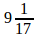hours

b)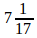hours

c)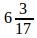hours

d)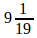hours

e)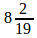hours

Q.14) A bag contains 3 white balls, 6 yellow balls and 4 blue balls. If one ball is taken at random, what is the probability that it is either blue or yellow?

a)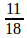b)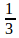c)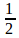d)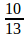e) 1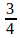Q.15) The sum of the four consecutive even numbers is 180, find the difference between the square of smallest and square of the largest even number.

a) 540

b) 480

c) 640

d) 720

e) 600

D.16-18) Find the approximate value of x

Q.16) 40.02% of 3599.9 + 34.9% of 2999.8 – 25% of 3000 = x

a) 1680

b) 1920

c) 1880

d) 1740

e) 1520

Q.17) ∛5812 + √1948 – √1511 = x2

a) 6

b) 25

c) 4

d) 35

e) 5

Q.18) 8889.8 + 8380.8 + 8009.9 + 2390.9 = x

a) 27922

b) 27432

c) 27732

d) 27422

e) 27672

Q.19) A is two times as efficient as B. A alone can do a piece of work in 4x days. If two of them together can do the same work in 8 days, then In how many days A alone can complete the whole work.

a) 24 days

b) 12 days

c) 18 days

d) 20 days

e) 36 days

Q.20) Find the sum of the prime numbers between 30 and 50.

a) 232

b) 238

c) 248

d) 152

e) 199

Q.1) a

=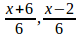X =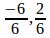=

X = -1, 0.333

=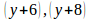Y = -6, -8.

So X > Y

Q.2) d

=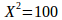X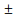10

Y =Y = 10

SoQ.3) e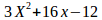=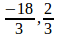Then X =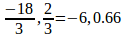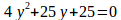=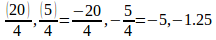So, the relationship cannot be determined.

Q.4) b

24x = 15y

So x = 15, y = 24.

So X < Y.

Q.5) a

17X – 32Y = -58

12X + 14Y = 142.

By solving the two equations, we get, X = 6, Y = 5.

So X > Y.

Q.6) a

From the question,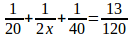2x = 30

X= 15

Q.7) d

Required probability,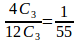Q.8) e

From the question,

Let length of platform be x metres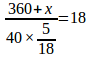X = 160 m

Q.9) b

After 5 hours

Man A covers 150 km

Therefore, man B covers 100 km

Required speed of man B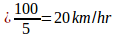Q.10) c

From the question,

A =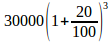A = Rs.51840

Q.11) b

Total age of 12 boys = 360 years

Total age of first 7 boys is 280 years

Total age of last six boys is 120 boys

Required age of 7th boy :

2x = 400 – 360 = 40 years

X = 20 years.

Q.12) e

From the question,

L×B = 72 m2

2(L+B) = 36m

L+B= 18.

Therefore Difference between L and B is 12-6 = 6m

Q.13) b

From the question,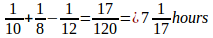Q.14) d

Required probability,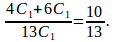Q.15) a

From the question,

X+ x+ 2+ x+ 4+ x+ 6 = 180

X= 42

Four even numbers are 42, 44, 46, 48

Required difference = 482 – 422 = 2304 – 1764= 540

Q.16) d

40% of 3600 + 35% of 3000 – 25% of 3000 = x

1440+ 1050 – 750= x

1740 = x

Q.17) e

18 + 44 – 39= x2

23 = x2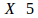Q.18) e

8890 + 8381 + 8010 + 2391 = x

X= 27672

Q.19) b

Efficient ratio of A to B = 2: 1

Time ratio of A to B = 1: 2

From the question,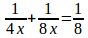X= 3

Hence, A alone can do the same work in 12 days

Q.20) e

Required total = 31 + 37+ 41 + 43 + 47 = 199.

We will post this Practice Questions – Daily till the start of the IBPS RRB PO & Assistant (Regularly)

Quantitative Aptitude @ 3:00 P.M

Reasoning Ability -@ 5:00 P.M

 QUANTITATIVE APTITUDE REASONING DAY 1 DAY 1 DAY 2 DAY 2 DAY 3 DAY 3 DAY 4 DAY 4 DAY 5 DAY 5 DAY 6 DAY 6 DAY 7 DAY 7 DAY 8 DAY 8 DAY 9 DAY 9 DAY 10 DAY 10 DAY 11 DAY 12 DAY 12

Attend GEEK TEST – SOLVE 10 Daily and enhance your preparation to the next level. Attend the Daily Tests with the Timer and improve your solving ability.

Also Please attend this BLUE WHALE QUESTION (which is a combination of many topics and combined into a single one). This is a One Stop Solution for all your Miscellaneous needs.

## BLUE WHALE CHALLENGE QUESTIONS

Get the FULL LENGTH MOCK TEST SERIES for SBI PO Mains 2018 , SBI CLERK Mains 2018 & BOB PO Mains 2018 from our Bankersdaily Store.